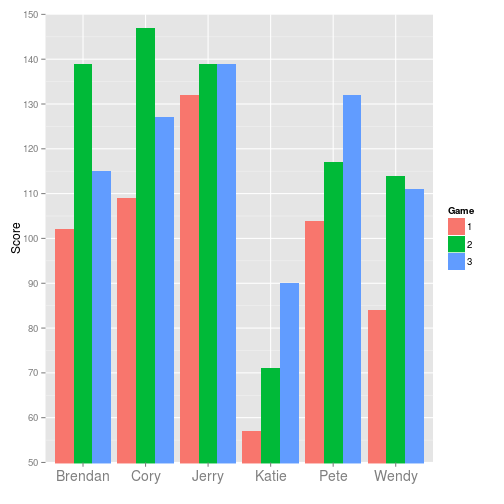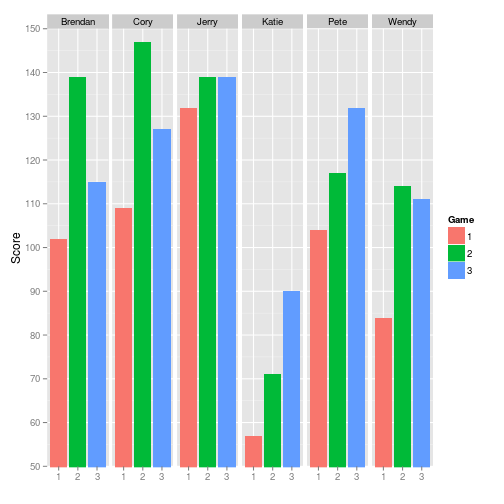## Wednesday, April 17, 2013

### ggplot dodged vs faceted bar chart

Updated: 7/9/2014

I've been bowling once per year at a charity event for the last few years and have kept track of the outcomes to share my group. I used ggplot2 to create a bar chart for the scores. Below are two graphs, one is dodged, the other is faceted. There's no right way here, but you can see the code and decide for yourself which is more aesthetically pleasing.

First is the dodged one…

``````library(ggplot2)
a <- 1:200

ggplot(df, aes(x=factor(name), y=score)) +
geom_bar(position="dodge", stat="identity",aes(fill=factor(game))) +
coord_cartesian(ylim=c(50, 150)) + scale_y_continuous(breaks=a[a%%10==0]) +
xlab("") + ylab("Score") + scale_fill_discrete(name="Game") +
theme(axis.text.x = element_text(size=14))
``````And next is the faceted one…

``````ggplot(df, aes(x=factor(game), y=score)) +
geom_bar(stat="identity",aes(fill=factor(game))) + facet_grid(.~name) +
coord_cartesian(ylim=c(50, 150)) + scale_y_continuous(breaks=a[a%%10==0]) +
scale_fill_discrete(name="Game") + xlab("") + ylab("Score")
``````The color is redundant in the faceted one, since there are labels for the game number. I kept the color in to make comparison with the dodged one easier.

Here's the code to produce these two graphs. The full code with the data to produce these plots and also the blog post using Rmarkdown is available in my github repo.